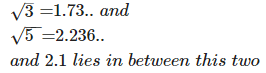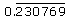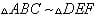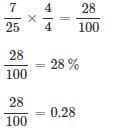Irrational Numbers - Number System

# Irrational Numbers - Number System

Test Description

## 25 Questions MCQ Test Advance Learner Course: Mathematics (Maths) Class 8 | Irrational Numbers - Number System

Irrational Numbers - Number System for Class 9 2022 is part of Advance Learner Course: Mathematics (Maths) Class 8 preparation. The Irrational Numbers - Number System questions and answers have been prepared according to the Class 9 exam syllabus.The Irrational Numbers - Number System MCQs are made for Class 9 2022 Exam. Find important definitions, questions, notes, meanings, examples, exercises, MCQs and online tests for Irrational Numbers - Number System below.
Solutions of Irrational Numbers - Number System questions in English are available as part of our Advance Learner Course: Mathematics (Maths) Class 8 for Class 9 & Irrational Numbers - Number System solutions in Hindi for Advance Learner Course: Mathematics (Maths) Class 8 course. Download more important topics, notes, lectures and mock test series for Class 9 Exam by signing up for free. Attempt Irrational Numbers - Number System | 25 questions in 25 minutes | Mock test for Class 9 preparation | Free important questions MCQ to study Advance Learner Course: Mathematics (Maths) Class 8 for Class 9 Exam | Download free PDF with solutions
 1 Crore+ students have signed up on EduRev. Have you?
Irrational Numbers - Number System - Question 1

### A number is irrational if and only if its decimal representation is:

Detailed Solution for Irrational Numbers - Number System - Question 1

A non-terminating, non-repeating decimal is a decimal number that continues endlessly, with no group of digits repeating endlessly. Decimals of this type cannot be represented as fractions, and as a result, are irrational numbers. Pi is a non-terminating, non-repeating decimal.

Irrational Numbers - Number System - Question 2

### The product or quotient of a non-zero rational number with an irrational number is:

Detailed Solution for Irrational Numbers - Number System - Question 2

The quotient of a non zero rational number with an irrational number will be irrational.

Irrational Numbers - Number System - Question 3

### A rational number between √3 and √5 is

Detailed Solution for Irrational Numbers - Number System - Question 3Irrational Numbers - Number System - Question 4

Which of the following statement about real numbers is false ?

Irrational Numbers - Number System - Question 5

(√12 + √10 - √2) is

Irrational Numbers - Number System - Question 6

The value obtained on simplifying (√5 + √6)2

Detailed Solution for Irrational Numbers - Number System - Question 6

(√5 + √6)2 = (√5)2 + (√6)2 + 2(√5)(√6)

= 5 + 6 +2√30

= 11 + 2√30

Irrational Numbers - Number System - Question 7

The decimal expansion 0.080080008000080000080000008……. is a

Irrational Numbers - Number System - Question 8

Sam bought 2+7⁄5 kg of flour in one week and 3+4⁄15 kg of flour in second week. The flour Sam bough altogether is

Detailed Solution for Irrational Numbers - Number System - Question 8

=2(7/5)+3(4/15)

=17/5+ 49/15

=(3×17 +49)/15

=(51+49)/15

=100/15

=20/3

Irrational Numbers - Number System - Question 9

An irrational number between 5/7 and 7/9 is :

Irrational Numbers - Number System - Question 10

Identify the irrational number among these.

Irrational Numbers - Number System - Question 11

On simplifying (√5 + √7)2 we get

Detailed Solution for Irrational Numbers - Number System - Question 11

(√5 + √7)2 = (√5)2 + (√7)2 + 2(√5)(√7)

= 5 + 7 + 2√35

= 12 + 2√35

Irrational Numbers - Number System - Question 12

The product of two numbers is -20/9. If one of the numbers is 4, find the other.

Irrational Numbers - Number System - Question 13

Which of the following is an irrational number ?

Irrational Numbers - Number System - Question 14

Insert one rational number between 2 and 3.​

Detailed Solution for Irrational Numbers - Number System - Question 14

A rational number between 2 and 3 =  2 + 3  / 2 = 2.5

An irrational number between 2 and 3 is 5 .

Irrational Numbers - Number System - Question 15= _______.

Irrational Numbers - Number System - Question 16

The ratio of the circumference of a circle to the diameter of the circle is.

Detailed Solution for Irrational Numbers - Number System - Question 16

Circles are all similar, and "the circumference divided by the diameter" produces the same value regardless of their radius. This value is the ratio of the circumference of a circle to its diameter and is called π (Pi). This constant appears in the calculation of the area of a circle and is a type of an irrational number known as a transcendental number that can be expressed neither by a fraction nor by any radical sign such as a square root, nor their combination.

Irrational Numbers - Number System - Question 17

Of the given numbers
(i) √27
(ii) √256
(iii) 0.3796
(iv) 7.478478…
(v) 1.101001000100001…

Irrational Numbers - Number System - Question 18

Which among these is the approximate value of π?

Detailed Solution for Irrational Numbers - Number System - Question 18

The number π  is a mathematical constant. Originally defined as the ratio of a circle's circumference to its diameter, it now has various equivalent definitions and appears in many formulas in all areas of mathematics and physics. It is approximately equal to 3.14159. It has been represented by the Greek letter "π" since the mid-18th century, though it is also sometimes spelled out as "pi". It is also called Archimedes' constant.

Irrational Numbers - Number System - Question 19

The decimal expansion 0.080080008000080000080000008….. is a

Detailed Solution for Irrational Numbers - Number System - Question 19

The decimal expansion 0.080080008000080000080000008….. is a. Non-terminating, non-recurring. Non-terminating, recurring.

Irrational Numbers - Number System - Question 20

How many rational numbers can you find between 5 and 6?​

Detailed Solution for Irrational Numbers - Number System - Question 20

Infinitely many. You can say 5.1 is a rational number lying between 5 and 6. 5.01, 5.001, 5.0001, 5.00001 and so forth.

Irrational Numbers - Number System - Question 21

Given that∠A = 50° ∠C = 35° ∠E = ?

Irrational Numbers - Number System - Question 22

Which of the following statements is false?

Irrational Numbers - Number System - Question 23

Convert 7/25 in the form of decimals,

Detailed Solution for Irrational Numbers - Number System - Question 23Irrational Numbers - Number System - Question 24

From the choices given below mark the co-prime numbers

Detailed Solution for Irrational Numbers - Number System - Question 24

The co prime numbers are 2,3 . Co means near prime means which cannot have a square root.

Irrational Numbers - Number System - Question 25

Which of the following statement is true?

Detailed Solution for Irrational Numbers - Number System - Question 25

The real numbers consist of both rational and irrational numbers. so the other three options are not true as the first one is not true because not only every real number is a rational number but the real number is rational and irrational both. same goes for the second one and the last option is not true because one half and the other half can be irrational or rational both.

## Advance Learner Course: Mathematics (Maths) Class 8

13 videos|61 docs|24 tests
 Use Code STAYHOME200 and get INR 200 additional OFF Use Coupon Code
Information about Irrational Numbers - Number System Page
In this test you can find the Exam questions for Irrational Numbers - Number System solved & explained in the simplest way possible. Besides giving Questions and answers for Irrational Numbers - Number System, EduRev gives you an ample number of Online tests for practice

## Advance Learner Course: Mathematics (Maths) Class 8

13 videos|61 docs|24 tests